利用UG软件进行盘形凸轮参数化建模技术研究与实现

1 序言

Unigraphics软件是一个集成化的CAD /CAECAM系统软件,它为工程设计人员提供了非常强大的应用工具,这些工具可以对产品进行设计 (包括零件设计和装配设计)、工程分析(有限元分析和运动机构分析)、工程图绘制、数控加工程序编制等,极大地提高了企业的技术创新能力和对市场的快速反应能力。UG NX基本工作流程是:设计人员先按照有关理论对零件造型 ,接着就可利用其数字化装配尽早发现问题 ,如检查干涉和间隙调整。利用其工程分析功能可以验证其运动学和动力学性能 ,据此可进一步完善设计。完善后的零件 ,一方面可自动转换为工程图以便加工 ,另一方面可根据需要 ,将一些复杂型面直接转换为数控加工程序。显然造型是第一步 ,也是比较重要的一步。

凸轮机构在现代机械中越来越广泛的得到应用，由于只需设计适当的轮廓，便可使从动件得到所需的运动规律，同时在某些机构中通常要求从动件的位移、速度或加速度按照给定的规律变化;若采用连杆机构实现给定的运动规律比较困难，特别是较复杂的运动规律很难实现;但凸轮机构能较易实现复杂运动规律，同时凸轮机构还具有结构简单、体积小等优点。

当前，在凸轮的设计过程中，利用计算机进行辅助设计已经成为主流，在UG的建模环境中利用UG表达式和规律曲线来快速准确的生成凸轮轮廓曲线，从而完成凸轮的三维实体建模。在装配环境中将凸轮与从动件按其匹配关系进行装配，从而实现凸轮机构的三维造型。在运动环境中，通过定义连杆、添加运动副对凸轮机构进行仿真运动和运动分析，通过仿真过程判断凸轮机构的运动结果是否与设计要求相一致，从而对凸轮机构进行改进。

2 盘形凸轮零件分析

图1是我公司生产某型号产品中的凸轮零件，偏心距为10mm，机构在运动过程中的运动规律为：当凸轮转过60°时，推杆等加速等减速上升10mm;凸轮继续转过120°推杆停止不动，凸轮再继续转过60°时，推杆等加速等减速下降10mm;最后，凸轮转过所余下120°时，推杆又停止不动。基圆半径为50mm，推杆滚子的半径为5mm。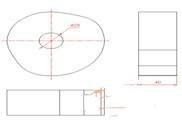图1 凸轮零件图

3 基于UG的凸轮参数化建模技术

参数化建模技术的思想是，利用数值驱动零件和部件的特征尺寸，在进行产品系列设计时，只需要添加多组数据即可;若要进行重新设计，只需要修改部分数据即可。

本文研究的盘形凸轮参数化建模技术，主要通过 UG 软件的表达式功能来实现。利用UG 软件进行偏置直动滚子推杆盘形凸轮零件实体造型设计的一般过程是：

(1) 提供参数。在设计之初，需要用户做的工作是，确定凸轮基圆半径r 0，选定推杆运动规律。

(2) 在UG建模环境中建立表达式。在 UG建模环境中，首先输入有明确赋值的变量和驱动参数，然后输入计算变量X、Y，建立控制凸轮轮廓曲线的表达式。

(3) 绘制凸轮理论轮廓曲线。完成表达式的建立以后，执行绘制规律曲线命令，以 t为系统变量，分别用定义的X、Y表示 UG坐标中的变量，将Z定义为常量0，即可得到需要的轮廓线段，所有曲线段构成完整的凸轮理论轮廓曲线。

(4) 绘制凸轮实际轮廓曲线。执行偏移曲线命令将理论轮廓曲线向内做按照滚子半径为距离的偏移，即可获得凸轮的实际轮廓曲线。

(5) 通过拉伸操作，获得凸轮的三维实体。将凸轮的实际轮廓曲线进行以厚度值为高度的拉伸操作，即可获得凸轮的三维实体。

通过上面5个步骤的具体讨论，可以看出，UG软件由于采用了表达式形式，从而避免了复杂的编程处理;通过偏移理论轮廓曲线，巧妙的获得了实际轮廓曲线，从而大大降低了工作量;通过改变其中的驱动参数或表达式值即可实现零件的相应改变，从而实现了完全的参数化建模。

4 实例凸轮的设计

本文采用解析法绘制盘形凸轮的实际轮廓曲线，计算出凸轮轮廓曲线上各点的坐标值，利用UG表达式及规律曲线实现凸轮的三维参数化建模。

4.1 凸轮轮廓曲线方程

在利用UG软件设计盘形凸轮之前，需要根据提供的参数推导出适合UG使用的表达式，而推导适合UG使用的表达式又需要根据实际情况明确给出盘形凸轮轮廓曲线的数学描述。若采用图解法设计盘形凸轮轮廓曲线其误差较大，对高速盘形凸轮或精度要求较高的盘形凸轮均不能满足设计要求，只能采用解析法进行设计，以达到高效、高精度的效果。

盘形凸轮机构轮廓曲线方程为：

x=(S0+S)sinδ+ecosδ

y=(S0+S)cosδ-esinδ

针对式中推杆的位移S，根据运动情况不同，各阶段计算如下：

4.1.1 推程阶段(δ01=60°)

等加速部分等减速部分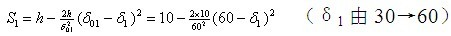4.1.2 远休止阶段(δ02=120°)

此时δ2由0→120，r=50+10=60mm，保持不变。

4.1.3 回程阶段(δ03=60°)

等加速部分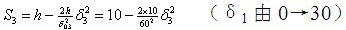等减速部分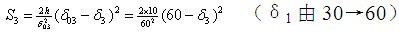4.1.4 近休止阶段(δ04=120°)

此时δ4由0→120，r=50mm，保持不变。

4.2 UG表达式的推导

4.2.1 已知条件，驱动参数

e=10(长度，mm)//偏心距

h=10(长度，mm)//升程;

R0=50(长度，mm)//基圆半径;

Rr=10(长度，mm)//滚子半径;

Angle01=60(角度，degree)//推程转角;

Angle02=120(角度，degree)//远休止角;

Angle03=60(角度，degree)//回程转角;

Angle04=120(角度，degree)//近休止角;

t=1//UG系统变量，无单位。

4.2.2 推程等加速阶段：Angle01=60

a1=0//起始角，角度，degree;

b1=30//终止角，角度，degree;

J1=a1*(1-t)+b1*t//中间角变量，角度，degree;

S1=2*h*J1*J1 /( Angle01* Angle01)//升程变量，长度，mm;

X1=(R0+S1)*sin(J1) +e*cos(J1)//理论轮廓曲线X坐标值，长度，mm;

Y1=(R0+S1)*cos(J1)-e*sin(J1) //理论轮廓曲线Y坐标值，长度，mm。

4.2.3 推程等减速阶段：Angle01=60

a2=30;b2=60;J2=a2*(1-t)+b2*t;Je2=60-J2;

S2=10-2*h*Je2*Je2 /( Angle01* Angle01);

X2=(R0+S2)*sin(J2) +e*cos(J2);

Y2=(R0+S2)*cos(J2) -e*sin(J2)

4.2.4 远休止阶段：Angle02=120

a3=60;b3=180;J3=a3*(1-t)+b3*t;S3=h;

X3=(R0+S1)*sin(J3) +e*cos(J3);

Y3=(R0+S1)*cos(J3) -e*sin(J3)。

4.2.5 回程等加速阶段：Angle03=60

a4=180; b4=210; J4=a4*(4-t)+b4*t; Je4=J4-180;

S4=h-2*h*Je4*Je4 /( Angle03* Angle03);

X4=(R0+S4)*sin(J4) +e*cos(J4);

Y4=(R0+S4)*cos(J4) -e*sin(J4)。

4.2.6 回程等减速阶段：Angle03=60

a5=210;b5=240;J5=a5*(5-t)+b5*t;

Je5=180+60-J5;

S5=2*h*Je5*Je5 /( Angle03* Angle03);

X5=(R0+S5)*sin(J5) +e*cos(J5);

Y5=(R0+S5)*cos(J5) -e*sin(J5)。

4.2.7 近休止阶段：Angle04=120

a6=240;b6=360;J6=a6*(6-t)+b6*t;S6=0;

X6=(R0+S6)*sin(J6) +e*cos(J6);

Y6=(R0+S6)*cos(J6) -e*sin(J6)。

4.3 基于UG的凸轮造型设计

(1)建立表达式方程。在UG建模环境下，输入4.2 中所推导的所有表达式。

(2)绘制凸轮理论轮廓曲线。参照前面说明执行绘制规律曲线命令，分别绘制各段曲线，最后形成完成的凸轮理论轮廓曲线，如图2所示。

(3)绘制凸轮实际轮廓曲线。执行偏置曲线命令，将凸轮理论轮廓曲线向内偏置，偏置距离为滚子半径10，结果如图3，此即为凸轮实际轮廓曲线。

(4)绘制凸轮中心孔。执行绘制圆弧命令，以原点为圆心，绘制一个直径为25 的圆。

(5)拉伸操作。选取凸轮实际轮廓曲线以及中心孔，执行拉伸命令，拉伸长度为凸轮的厚度40。结果如图4 所示。此即为要求设计的凸轮实体。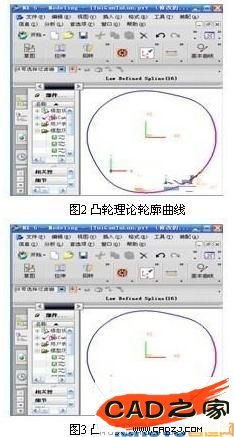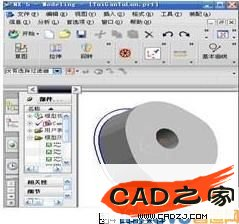此时如果已知条件发生改变，则不需要全部重来，只需改变表达式的值，凸轮轮廓曲线及相应的实体都会随之改变。UG软件参数化建模技术的优势即在如此。

5 结论

本文提供的盘形凸轮参数化建模方法是一个通用方法，不仅工作量小、简便易学，而且设计精度高。通过改变相关变量，可以满足不同用户对盘形凸轮设计的要求。

利用本文所提供的UG参数化建模技术，可以极大地缩短凸轮的设计制造周期，在生产实践中具有显著的实用价值和经济效益，并为以后进行凸轮运动分析、动力分析、有限元分析、虚拟装配等，都打下了良好的基础。